# Multiplying Decimals

Multiplying Decimals• We ignore the decimal points and any zeros in front of them and then multiply.
• So 0.3 × 0.5 can be thought of as 3 × 5, which equals 15.
• 3 is ten times larger than 0.3 and 5 is ten times larger than 0.5, so our answer is 100 times too big.
• We divide 15 by 100 by moving the decimal point two places.
• We then write a zero in front of the decimal point if there is no digit in front of it.
• 3 × 5 = 15 and so, 0.3 × 0.5 = 0.15 .
• 0.3 has one decimal place and 0.5 has one decimal place, which is two decimal places in total.
• Our answer of 0.15 also has two decimal places.

Multiply the decimals like whole numbers.

Move the decimal point so that the answer has the same number of decimal places as the numbers in the question combined.• We ignore the decimal points and the zeros in front for the decimal numbers.
• We think of 2.1 as 21 and 0.3 as 3.
• We multiply each digit in 21 by 3 to get 63.
• Now we correct the answer by counting the decimal places in the question.
• 2.1 has one decimal place and 0.3 has one decimal place.
• There are 2 decimal places in the question so there will be 2 decimal places in the answer.
• We put 2 decimal places after the decimal point and fill in our answer from right to left.
• We put a zero in front of the decimal point.
• 2.1 × 0.3 = 0.63.#### Multiplying Decimals using Long Multiplication#### Multiplying by Decimals Interactive Question Generator

Multiplying Decimals with Times Tables: Interactive Questions

# How to Multiply Decimals

To multiply decimals use the following steps:

1. Ignore the decimal points then multiply the numbers that remain.
2. Count the number of decimal places in all of the numbers of the question combined.
3. Place the decimal point so that there are the same number of decimal places in the answer and the question.
4. Add a zero in front of the decimal point if there are no digits there.

Here is an example of how to use these steps to multiply two decimal numbers.

We have 1.1 × 0.8.

Step 1: Ignore the decimal points then multiply the numbers that remain.

Ignoring the decimal point in 1.1, we have 11.

Ignoring the decimal point and the zero before it in 0.8, we have 8.

We multiply 11 × 8.From our times tables, we know that 11 × 8 = 88.

Step 2: Count the number of decimal places in all of the numbers of the question combined.

The number of decimal places in each number is equal to the number of digits to the right of the decimal point. The decimal places are shown underlined in each number below.

1.1 has one decimal place.

0.8 has one decimal place.

In total, the question has two decimal places. One from 1.1 and one from 0.8.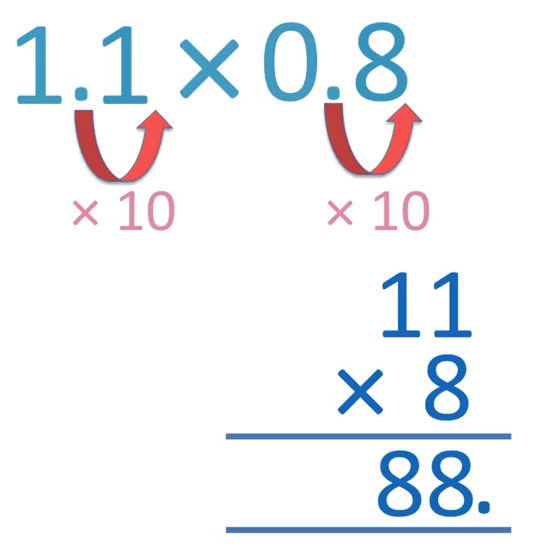The number of decimal places in each number is also equal to the number of digits that the decimal point must jump over to get to the right end of the number.

We can see that we have one jump shown in each number and that there are 2 jumps in total in both numbers of our question. There are 2 decimal places in total in our question.

Step 3: Place the decimal point so that there are the same number of decimal places in the answer and the question.

We know that there are 2 decimal places in the question and so, there must be 2 decimal places in our answer.

It is easiest to first write the decimal point and then count the 2 decimal places to the right of this.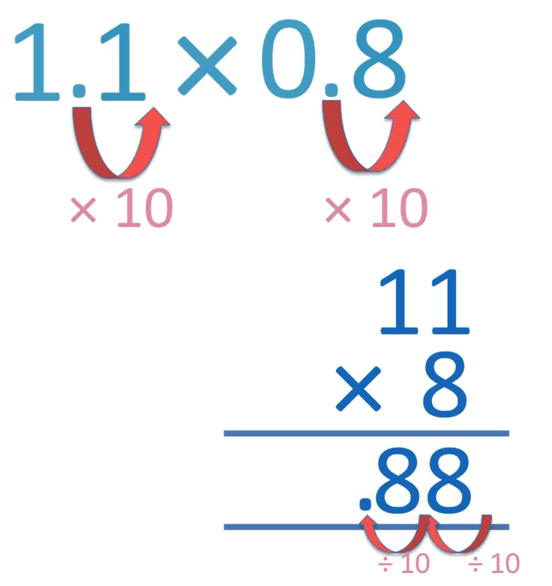We work from right to left placing the digits of our answer in.

We place the two 8 digits in the two decimal places to the right of the decimal point.

Step 4: Add a zero in front of the decimal point if there are no digits there.

We have .88 and so, there are no digits in front of the decimal point.

We must write a zero in front of the decimal point to show that there are no units there.The answer to 1.1 × 8 = 0.88.## Why the Trick for Multiplying Decimals Works

In this example we have 0.4 × 0.3.

We think of it as 4 × 3, which equals 12.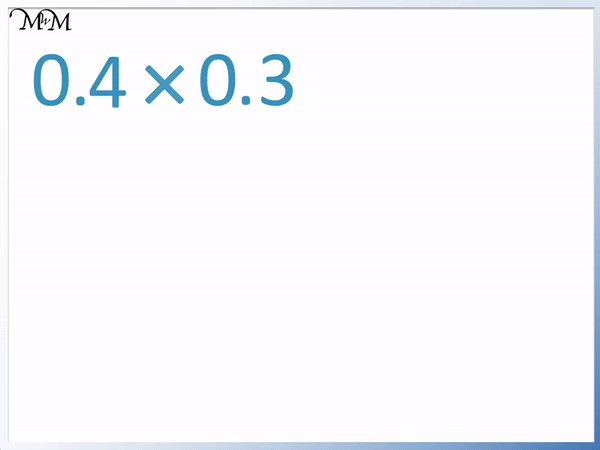We have made 0.4 ten times bigger to get 4 and 0.3 ten times bigger to get 3.

The answer of 12 is 100 times too big and so we need to make it 100 times smaller by moving the decimal point two places left.

We get .12 and we write a zero in front to get 0.12 as our answer.

To make each decimal in the question into a whole number, the decimal point must move past each decimal place.

Each decimal place in the question is an extra division by 10.

We can simply count the number of decimal places in all numbers of the question and make sure that there are the same in the answer.

0.4 has 1 decimal place and 0.3 has 1 decimal place.Our answer has 2 decimal places and so, we can put two lines as decimal places after the decimal point. We then fill these places with the answer to 4 × 3.

We work from right to left, so 2 goes in on the rightmost decimal place and 1 goes in the left one.

There are no more digits left so we just write a zero in front of the decimal point.

## Examples of Multiplying Decimals by Decimals

In this example we have 0.2 × 4.

We think of the multiplication as 2 × 4, which is equal to 8.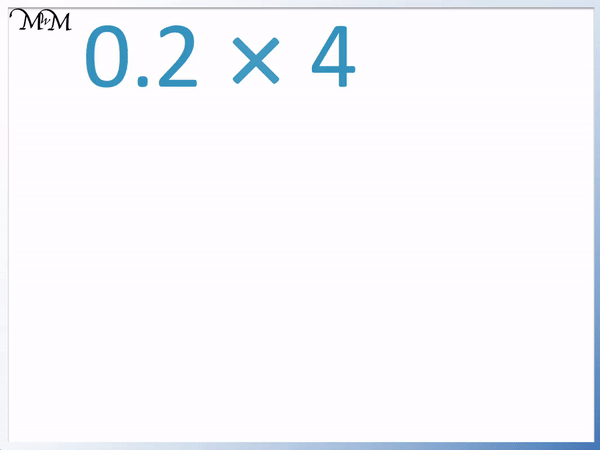However this answer is ten times too big because we had 0.2 rather than 2. We made 0.2 ten times larger to get this answer.

We have to divide by ten to undo this. We move the decimal point one place left and write a zero in front of it.

0.2 × 4 = 0.8

We can see that 0.2 has 1 decimal place and so does our answer of 0.8.

In this next example of multiplying decimals we have 0.3 × 0.5.

We ignore the decimal points and the zeros in front of them and work out 3 × 5.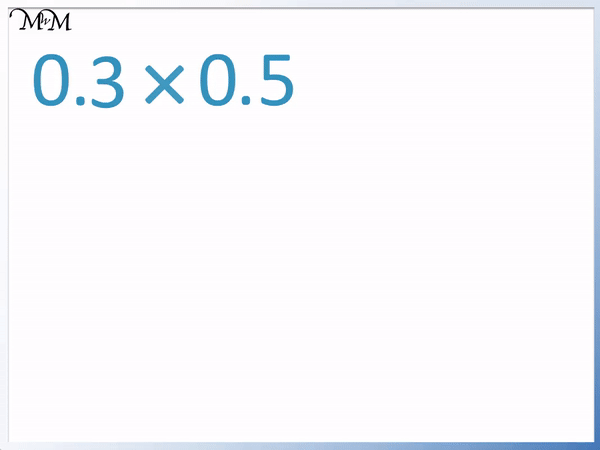3 × 5 = 15, however we multiplied 0.3 by ten to get 3 and we multiplied 0.5 by ten to get 5.

So we must divide 15 by ten and then by ten again to get our correct answer.

We can see that there is one decimal place in 0.3 and one decimal place in 0.5. This makes 2 decimal places in total in our question.

We know that our answer must have two decimal places after the decimal point.

0.3 × 0.5 = 0.15

## Multiplying Decimals with Zeros in the Product

If the product in a decimal multiplication ends in any zeros, then remove the zeros before writing the final answer.

Here is an example of a decimal multiplication in which we must remove zeros from our final answer.

We have 0.25 × 0.04.

We first work out 25 × 4, which equals 100.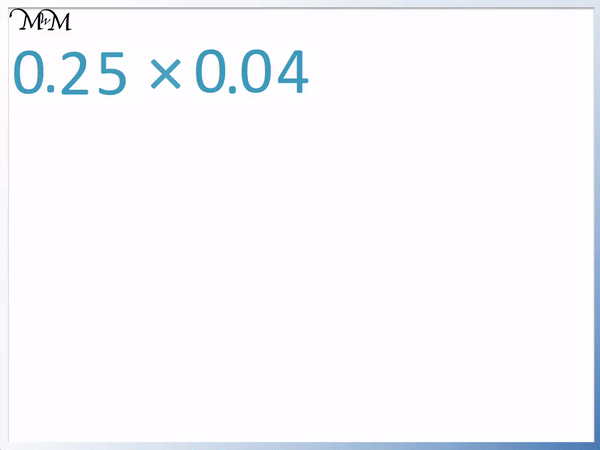We have 2 decimal places in 0.25 and 2 decimal places in 0.04

Our answer will have a total of 4 decimal places.

We have 0.0100, which has 4 decimal digits: 0100.

However, we do not need to write the last two zeros that follow the 1.

0.0100 is the same as 0.01.

0.25 × 0.04 = 0.01

In this example we have 3.4 × 7.5.

We ignore the decimal points and work out 34 × 75.

This is quite a large calculation and so we can use long multiplication to work out the answer on grid paper.

First we multiply the digits in 34 by 5.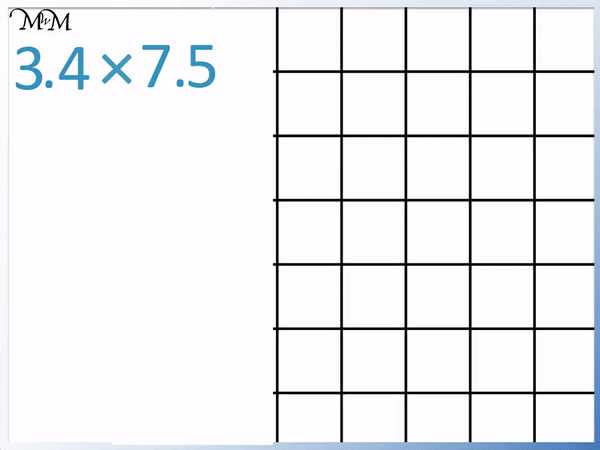4 × 5 = 20, so we write the 0 and carry the 2.

3 × 5 = 15 and we add the 2 that we carried to make 17.

34 × 5 = 170.

We now multiply the digits in 34 by 70.

To do this we write a zero to multiply by ten and then multiply by 7.4 × 7 = 28, so we write the 8 and carry the 2.

3 × 7 = 21 and we add the 2 that we carried to make 23.

34 × 70 = 2380

We now add the digits to get the answer to 34 × 75.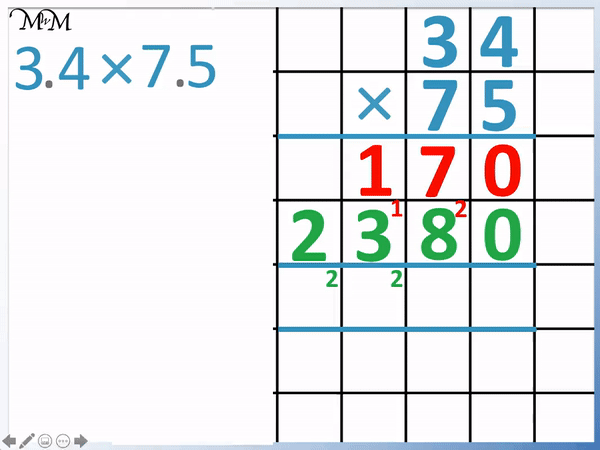34 × 75 = 2550

We now correct our answer to obtain the correct answer to the decimal multiplication of 3.4 × 7.5.

We have 1 decimal place in 3.4 and 1 decimal place in 7.5. There are 2 decimal places in total in the question and so we will have 2 decimal places in the answer.

3.4 × 7.5 = 25.50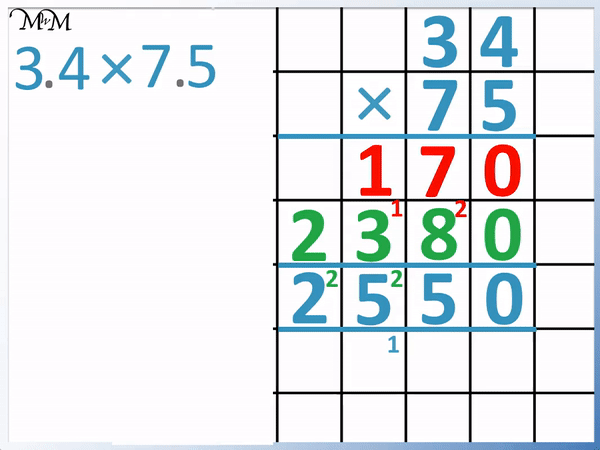Now that we have got our answer, we can see that there is a zero at the end of the answer. Remember that we can remove any zeros at the end of a decimal number.

25.50 is the same as 25.5 and so, our answer is 25.5.

## Multiplying Larger Decimals using Grid Paper

In these examples we must use long multiplication on grid paper to work out the answers to the decimal multiplications.

In this example we have 5.2 × 1.6.

We first multiply 52 and 16. We multiply the digits in 52 by 6.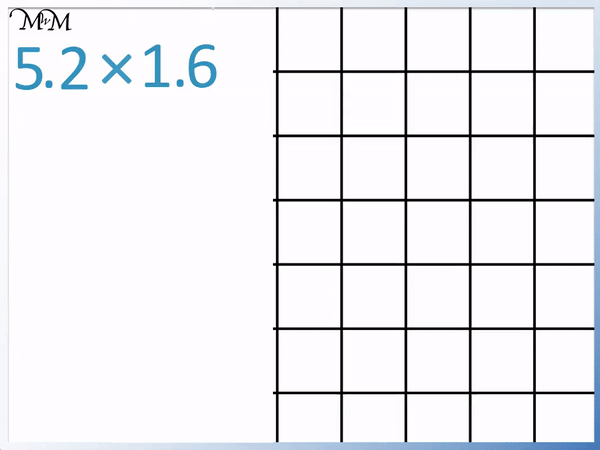2 × 6 = 12 so we write the 2 and carry the 1 ten.

Next we multiply 5 by 6 which equals 30. We add the 1 that we carried to make 31.

52 × 6 = 312

We now multiply the digits in 52 by 10. 52 × 10 = 520.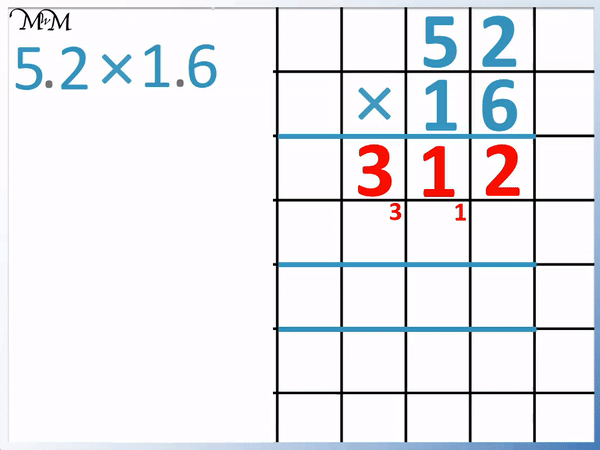We now add the digits in each column to get our answer .52 × 16 = 832

Finally, we make sure that our answer has the same number of decimal places as in the question.

5.2 has 1 decimal place and 1.6 has 1 decimal place. The question has 2 decimal places so our answer will have 2 decimal places.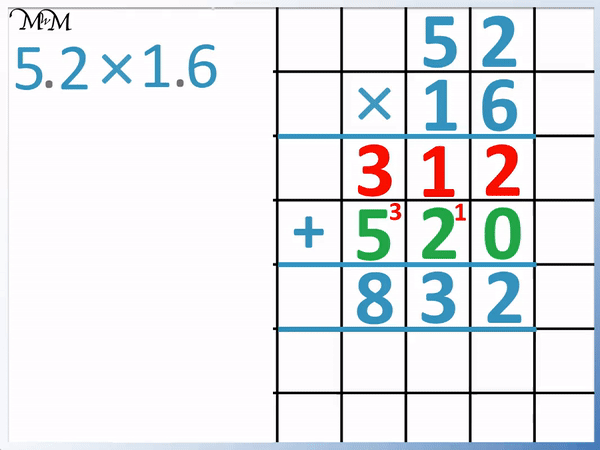We write the ‘3’ and the ‘2’ of 832 in the two decimal places of the answer and the ‘8’ must go on the left of the decimal point.

The digits in our answer of 8.32 must be in the same order as in 832.

In this example we have 6.3 × 7.02.

We multiply 63 by 702 using long multiplication.

63 × 2 = 126Next we multiply 63 by 0.

Anything times zero equals zero and so, we do not need to write anything for this.Next we multiply the digits in 63 by 700. We first multiply by 100 by writing two zeros and then we can simply multiply by 7.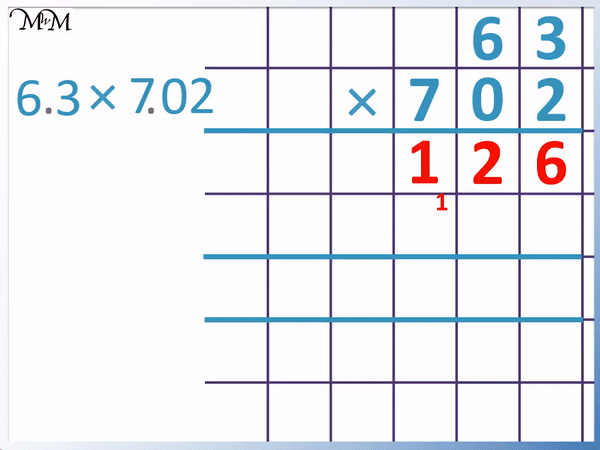3 × 7 = 21, so we write the 1 and carry the 2 tens.

6 × 7 = 42, plus the 2 we carried equals 44.

63 × 700 = 44100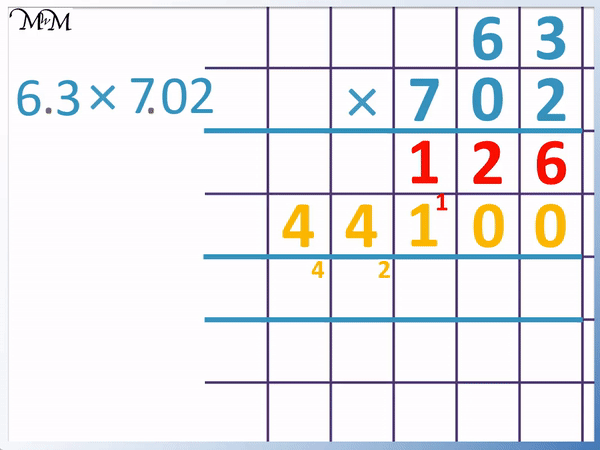We add the digits to get our answer to 63 × 702, which is 44226.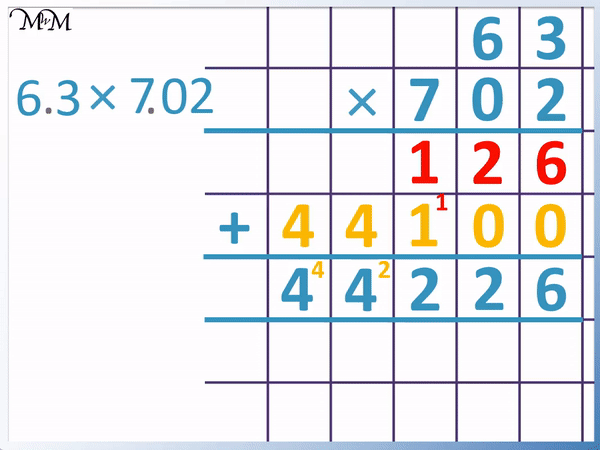We now use our decimal place trick to convert our answer to 63 × 702 into the correct answer for 6.3 × 7.02.

6.3 has 1 decimal place and 7.02 has 2 decimal places.

This is three decimal places in total and so, our answer must have three decimal places.

We put the digits of 226 in the three decimal place spots and then the 44 goes in front of the decimal point.Now try our lesson on Finding Simple Percentages of Amounts: 10%, 25%, 50% and 100% where we learn how to find simple percentages of amounts.error: Content is protected !!